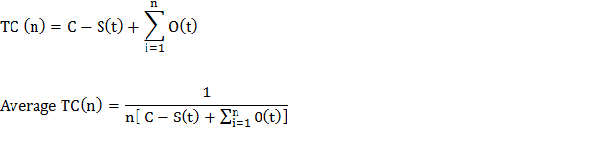Replacement of Goods Which Degrades with Time Without Considering Any Change in Value of Money

With every passing operating period, most of the parts of machinery get deteriorated. The costs involved in its  repair and maintenance continues to grow as time passes. A certain stage or time comes when on calculation it is found out that the total cost involved in its repair is more than the price of a new equipment.

If explained in a simplified way, assume that you have a car which is nearly worn out. On an overall analysis, you find out that the total amount which you are investing on its maintenance is too high. And on comparison, you find the value of a new car is less that the old one’s maintenance cost. So the obvious economic solution for you is the replacement of the old car with a new one.

The concept is explained in the form of a formula.

TC (n) = C – S(t) +

Average TC (n) =

Where,

TC (n) = total cost utilised for ‘n’ years on an equipment.

C = an equipment’s capital cost

S (t) = an equipment’s scrap value after being used for ‘t’ years

N = number of years when an equipment can be used

O (t) = cost involved in the maintenance and operation of a machinery at time ‘t’

t = in this case, it depicts time which is a discrete variableAccording to this equation, a certain machinery can be utilised for a certain number of years as long as average TC (n) is kept minimum. The most economic year (ith) for equipment replacement is considered when the average total cost keeps dropping up to ith year and then it starts to rise from (i + 1) year.

One should also understand the idea of depreciation cost involved here.Cost associated with equipment continues to decrease with time. It is with the help of commonly used two methods, calculation of decreasing costs of equipment can be calculated. The 2 methods are:

• Diminishing value method
• Straight line depreciation method### Customer Reviews

My Homework Help
Rated 5.0 out of 5 based on 510 customer reviews at